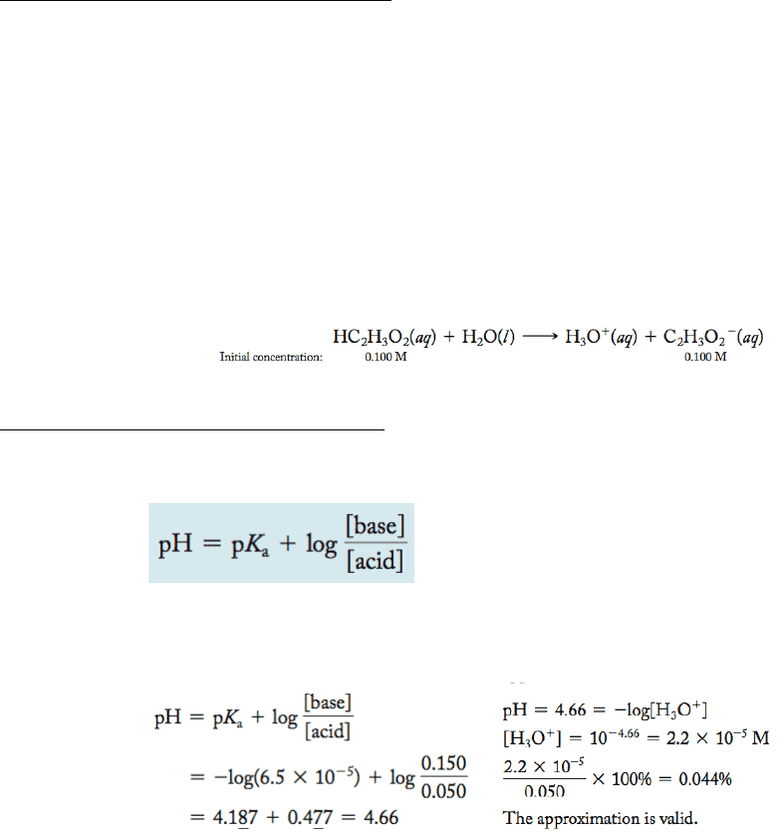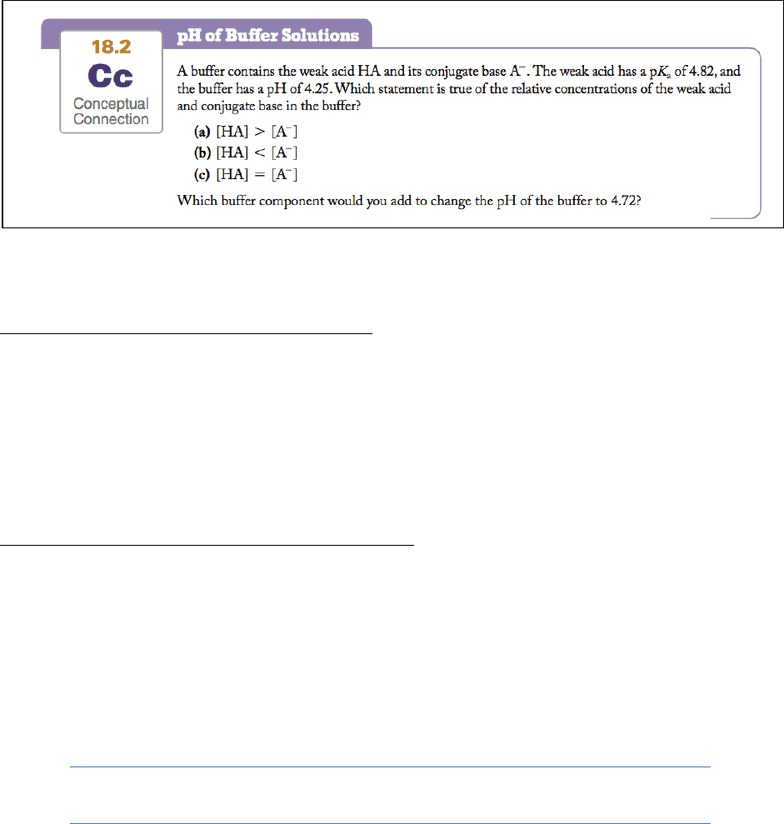# CHEM 1C Chapter Notes - Chapter 18: Silver Nitrate, Sodium Hydroxide, Lewis Acids And Bases

140 views18 pages
School
UC-Irvine
Department
Chemistry
Course
CHEM 1C
ProfessorChapter 18: Aqueous Ionic Equilibrium
18.1 The Danger of Antifreeze
§ Dogs/cat dies from consuming common household items of antifreeze
o Antifreeze is an aqueous solution of ethylene glycol
o It’s sweet and acts on the brain like alcohol (compound is alcohol)
o In the liver, it’s oxidized to an acid and goes into the blood. The acidity of the blood
is important because pH has a narrow pH range it can function in.
§ This nearly constant blood pH is maintained by buffers. Buffers resist pH
changes by neutralizing added acid or base
18.2 Buffers: Solutions That Resist pH Change
§ When acid/base is added most solutions significantly change
§ However, buffers resist pH change by neutralizing added acid or added base. Buffer
contains either:
o 1. Significant amounts of both a weak acid and its conjugate base
o 2. Significant amounts of both a weak base and its conjugate acid
§ Blood: carbonic acid and it’s conjugate base
o When additional base is added to a buffer, the weak acid reacts with the base,
neutralizing it.
o When additional acid is added to a buffer, the conjugate base reacts with the acid,
neutralizing it
o A weak acid alone does not ionize enough to form conj. base so it does not contain
sufficient base to be a buffer
§ Same thing with a weak base, it doesn’t have enough acid to be a buffer
o A buffer must contain significant amounts of both weak acid and its conj. base
(same with weak base)
§ As long as the amount added of a base/acid is less than the amount of
base/acid in the solution, the buffer will be able to neutralize it
§ Consider the simple buffer made by dissolving acetic acid (HC2H3O2) and sodium
acetate (NaC2H3O2) in water (C2H3O2 - is the conjugate base of HC2H3O2)
Unlock document

This preview shows pages 1-3 of the document.
Unlock all 18 pages and 3 million more documents.o Add strong base: NaOH(aq) + HC2H3O2(aq) à H2O(l) + NaC2H3O2(aq)
o Add strong acid: HCl(aq) + NaC2H3O2(aq) à HC2H3O2(aq) + NaCl(aq)
§ Base reacts with conj. acid and acid reacts with conj. base
o The weak acid neutralizes added base
o The weak base neutralizes added acid
Calculating the pH of a Buffer Solution
§ Common Ion effect: Adding a conjugate lessons the effect of ionization
o If we add a conj., according to Le Chatelier’s principle, the reaction shifts to the left
so that less of the acid is ionized. In other words, the presence of an ion causes the
acid to ionize even less than it normally would resulting in a less acidic solution
(higher pH).
o Named common ion effect because the solution contains two substances
(HC2H3O2 and NaC2H3O2) that share a common ion (C2H3O2- )
§ Consider a solution that initially contains HC2H3O2 and NaC2H3O2, each
at a concentration of 0.100 M. The acetic acid ionizes according to the
reaction:
The Henderson–Hasselbalch Equation
§ Can use moles only in this equation when filling in for the base and acid
§ Calculate the pH of a buffer solution that is 0.050 M in benzoic acid (HC7H5O2) and
0.150 M in sodium benzoate (NaC7H5O2). For benzoic acid, Ka = 6.5 * 10-5
§ To use small approximation both must be true
o Initial conc. of acids (and/or bases) are not too dilute
§ For buffers, initial conc. of acids and conj. Bases should be at least 102-103
times greater than the equilibrium constant
o The equilibrium constant is fairly small
Unlock document

This preview shows pages 1-3 of the document.
Unlock all 18 pages and 3 million more documents.o Answer: pH of buffer is greater than the pKa thus, there is more acid. A. Add more
weak base if you want to make the pH higher (more basic)
Calculating pH Changes in a Buffer Solution
§ 1. The stoichiometry calculation in which we calculate how the addition changes the
relative amounts of acid and conjugate base. Calculate changes in amounts in moles of
buffer components when you add the acid or base
§ 2. The equilibrium calculation in which we calculate the pH based on the new amounts
of the conjugate base
o use ICE chart or pH=pka+log[base/acid]
Buffers Containing a Base and Its Conjugate Acid
§ same thing recall:
o pka + pkb = 14
o Ka x Kb =Kw
18.3 Buffer Effectiveness: Buffer Range and Buffer Capacity
§ Buffers can be destroyed if you add too much acid or base
§ In this section, we examine two such factors: the relative amounts of the acid and conjugate base
and the absolute concentrations of the acid and conjugate base.
§ We then define the capacity of a buffer (how much added acid or base it can effectively
neutralize) and the range of a buffer (the pH range over which a particular acid and its
conjugate base can be effective).
Unlock document

This preview shows pages 1-3 of the document.
Unlock all 18 pages and 3 million more documents.